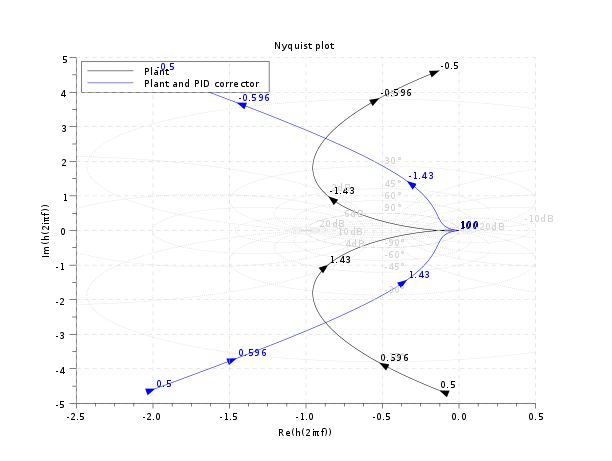Scilab Home page | Wiki | Bug tracker | Forge | Mailing list archives | ATOMS | File exchange
Change language to: English - Português - 日本語

Please note that the recommended version of Scilab is 6.1.1. This page might be outdated.
See the recommended documentation of this function

Manuel Scilab >> CACSD > nyquist

# nyquist

nyquist plot

### Calling Sequence

```nyquist( sl,[fmin,fmax] [,step] [,comments] )
nyquist( sl, frq [,comments] )

### Arguments

sl

a continuous or discrete time SIMO linear dynamical system ( see: syslin).

fmin,fmax

real scalars (frequency bounds (in Hz))

step

real (logarithmic discretization step)

string vector (captions).

frq

vector or matrix of frequencies (in Hz) (one row for each output of `sl`).

db,phi

real matrices of modulus (in dB) and phases (in degree) (one row for each output of `sl`).

repf

matrix of complex numbers. Frequency response (one row for aech output of `sl`)

### Description

Nyquist plot i.e Imaginary part versus Real part of the frequency response of `sl`.

For continuous time systems `sl(2*%i*%pi*w)` is plotted. For discrete time system or discretized systems `sl(exp(2*%i*%pi*w*fd)` is used ( `fd=1` for discrete time systems and `fd=sl('dt')` for discretized systems )

`sl` can be a continuous-time or discrete-time SIMO system (see `syslin`). In case of multi-output the outputs are plotted with different symbols.

The frequencies are given by the bounds `fmin,fmax` (in Hz) or by a row-vector (or a matrix for multi-output) `frq`.

`step` is the ( logarithmic ) discretization step. (see `calfrq` for the choice of default value).

`comments` is a vector of character strings (captions).

`db,phi` are the matrices of modulus (in Db) and phases (in degrees). (One row for each response).

`repf` is a matrix of complex numbers. One row for each response.

Default values for `fmin` and `fmax` are `1.d-3`, `1.d+3` if `sl` is continuous-time or `1.d-3`, `0.5`/sl.dt (nyquist frequency) if `sl` is discrete-time.

Automatic discretization of frequencies is made by calfrq.

To obtain the value of the frequency at a selected point(s) you can activate the datatips manager and click the desired point on the nyquist curve(s).

### Graphics entities organization

The `nyquist` function creates a compound object for each SISO system. The following piece of code allows to get the handle on the compound object of the ith system:

```ax=gca();//handle on current axes
hi=ax.children(\$+i-1)// the handle on the compound object of the ith system```

This compound object has two children: a compound object that defines the small arrows (a compound of small polylines) and the curve labels (a compound of texts) and a polyline which is the curve itself. The following piece of code shows how one can customize a particular nyquist curve display.

```hi.children(1).visible='off'; //hides the arrows and labels
hi.children(2).thickness=2; //make the curve thicker```

### Examples

```//Nyquist curve
s=poly(0,'s')
h=syslin('c',(s^2+2*0.9*10*s+100)/(s^2+2*0.3*10.1*s+102.01));
h1=h*syslin('c',(s^2+2*0.1*15.1*s+228.01)/(s^2+2*0.9*15*s+225))
clf();    nyquist(h1)
// add a datatip
ax=gca();
h_h=ax.children(\$).children(2);//handle on Nyquist curve of h
tip=datatipCreate(h_h,[1.331,0.684]);
datatipSetOrientation(tip,"upper left");``````//Hall chart as a grid for nyquist
s=poly(0,'s');
Plant=syslin('c',16000/((s+1)*(s+10)*(s+100)));
//two degree of freedom PID
tau=0.2;xsi=1.2;
PID=syslin('c',(1/(2*xsi*tau*s))*(1+2*xsi*tau*s+tau^2*s^2));
clf();
nyquist([Plant;Plant*PID],0.5,100,["Plant";"Plant and PID corrector"]);
hallchart(colors=color('light gray')*[1 1])
//move the caption in the lower rigth corner
ax=gca();Leg=ax.children(1);
Leg.legend_location="in_upper_left";```